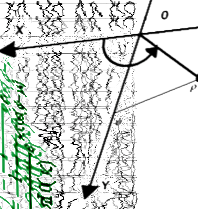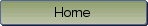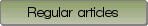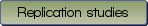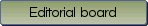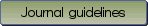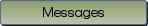Le traitement statistique des proportions incluant l'analyse de variance, avec des exemples // The statistical handling of proportions including analysis of variance, with worked out examples

Bibliographic information: BibTEX format RIS format XML format APA style
Cited references information: BibTEX format APA style

Laurencelle, Louis
272-285
Keywords: Proportions , Transformation angulaire , Proportions corrélées , Analyse de variance , Test de McNemar , Angular transformation , Correlated proportions , Analysis of variance , McNemar's test
(no sample data)   (Appendix)

The simple proportion, $p = x / n$, a pervasive statistical tool equally used in public and academic research areas, is in the literature still short of the necessary analytical implements needed for full-scale statistical treatments. This is partly ascribable to its discrete numerical character, but it proceeds mostly from its other distributional properties: its variance is tied up with its expectation, and its density shows a strong U-shaped asymmetry reactive to its $\pi$ parameter, the habitual normal-based analytical procedures thus being contra-indicated. We here revive the Fisher-Yates angular transformation of the proportion, $y\left ( {x,n} \right ) = si{n^{ - 1}}\sqrt x$, heralded for its $\pi$-independent variance and smoothed-out non-normality, and put to trial three of its improved descendants (Anscombe 1948, Tukey \& Freeman 1950, Chanter 1975). Following a would-be thorough study of the three $y$ functions retained (bias, variance, precision, test accuracy, power), largely documented in Laurencelle's (2021a) investigation, we develop and illustrate the $z$ test of significance on one proportion, the $z$ test on the difference of two independent proportions, the analysis of variance of $k \ge 2$ independent proportions and finally the test of two and anova of $k$ correlated proportions. A critical appraisal of the McNemar test for the difference of two correlated proportions is also essayed.

Be informed of the upcoming issues with RSS feed:RSS